Get instant live expert help with Excel or Google Sheets“My Excelchat expert helped me in less than 20 minutes, saving me what would have been 5 hours of work!”

#### Post your problem and you’ll get expert help in seconds.

Your message must be at least 40 characters
Our professional experts are available now. Your privacy is guaranteed.

# How to Convert Letters to Numbers And Vice Versa – Excelchat

Excel enables us to convert letters to numbers and numbers to letters. In this tutorial, we will learn how to convert letters to numbers and vice versa using the SUBSTITUTE function, COLUMN function, and VBA.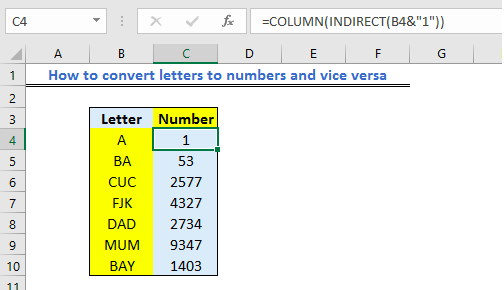Figure 1 – Example of how to convert column letter to a number

## How to Convert Column Letter to number

• We will prepare our table as shown in the figure below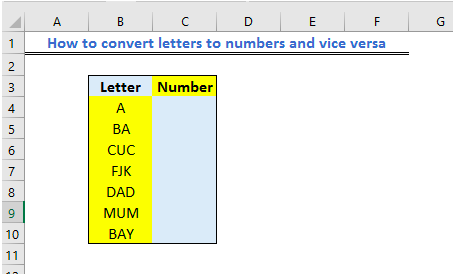Figure 2 – Letter to number

• We will highlight Cell C4 and insert the formula below in the formula bar

`=COLUMN(INDIRECT(B4&"1"))`

• We will press the Enter Key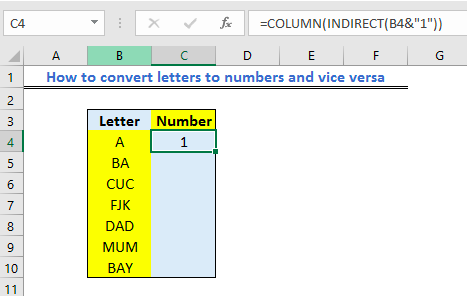Figure 3 – Using COLUMN Function to convert letters to a number

• We will click on Cell C4 and drag down the fill handle tool to copy the formula down the column.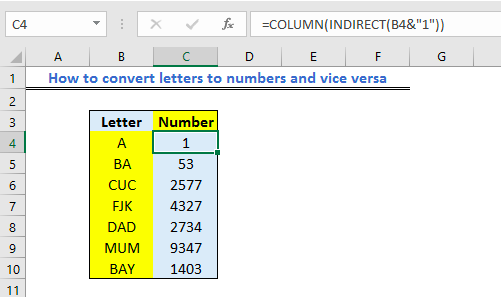Figure 4 – Column letter to a number

## Using VBA to Convert Column Letter to a Column Number

• Again, we will prepare a table as shown in the figure below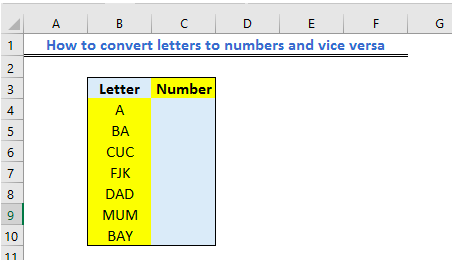Figure 5 – Setting up data to convert letters to numbers

• We will press ALT + F11 to open the Microsoft Visual Basic Applications window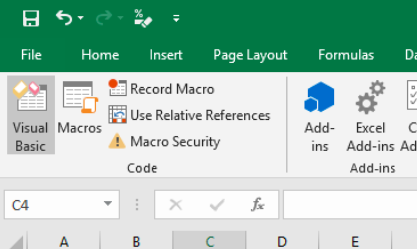Figure 6 – Using VBA to convert column letter

• Next, we will go to Insert and Select Module
• In the blank space, we will enter the formula below:

`Public Function ToColNum(ColN)`

`    ToColNum = Range(ColN & 1).Column`

`End Function`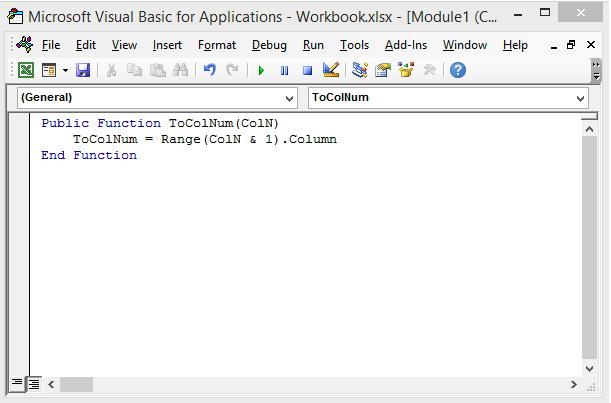Figure 7 – Convert column letter to number using VBA

• We will save the code and go back to the worksheet
• We will enter the formula below in Cell C4

`=Tocolnum("A")`

## Where A is the content of Cell B4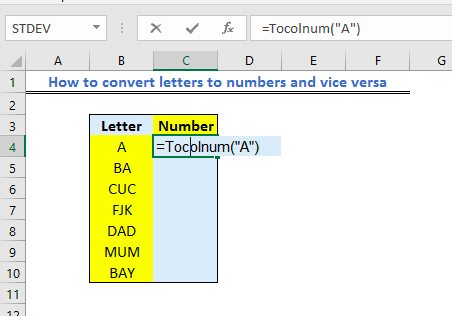Figure 8 – Return with column letter in excel

• We will press Enter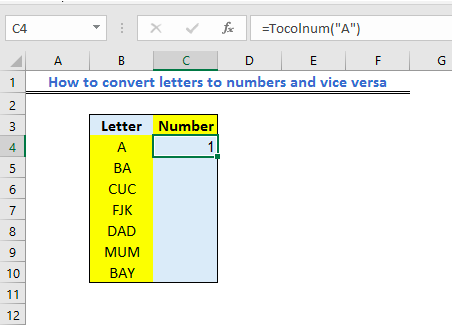Figure 9 – Excel column letter to a number

• Again, to get the column value for the content of Cell C5, we will enter the formula

`=Tocolnum("BA")`

## Where BA is the content of Cell B5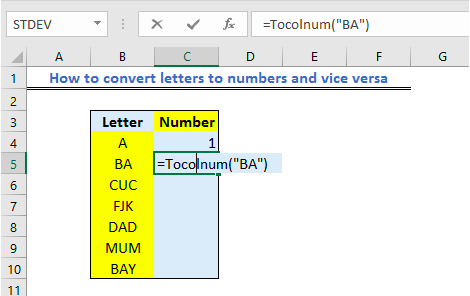Figure 10 – Letter to the column number

• We will press the Enter key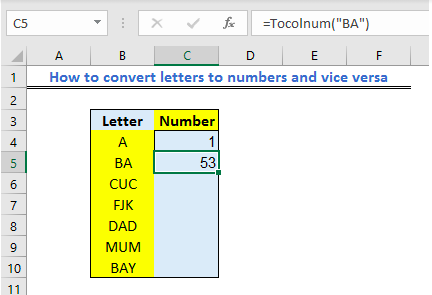Figure 11 – Excel column letter to a number

• We will have this result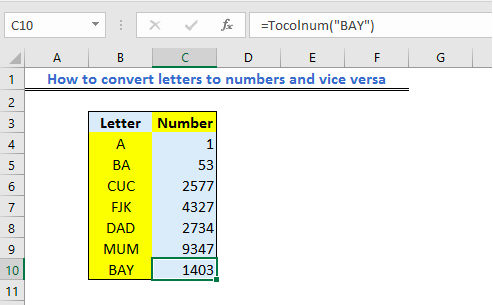Figure 12 – Excel returns with a column number

## How to Convert Column Number to Column Letter

• We will prepare our table as shown in the figure below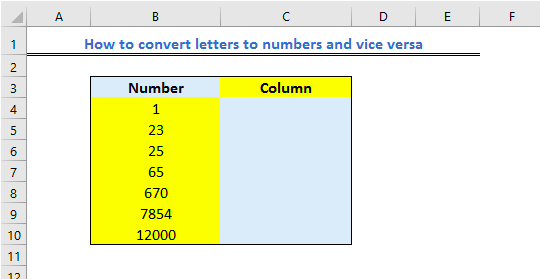Figure 13 – Setting up data for converting column number to letter

• We will click on Cell C4 and insert the formula below in the formula bar

`=SUBSTITUTE(ADDRESS(1,B4,4),"1","")`

• We will press the Enter Key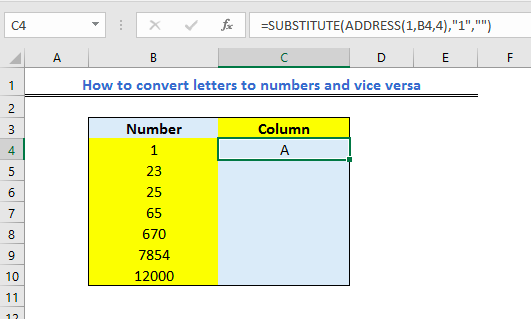Figure 14 – Convert column number to letter

• We will click again on Cell C4. Next, we will use the fill handle tool to drag down the formula into other cells in Column C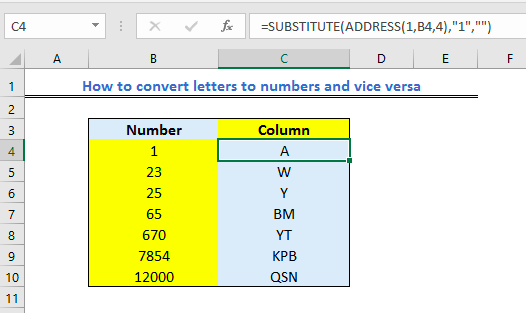Figure 15 – Excel returns with column letter

## How to Convert Column Number to Column Letter Using VBA

• We will create a table as shown below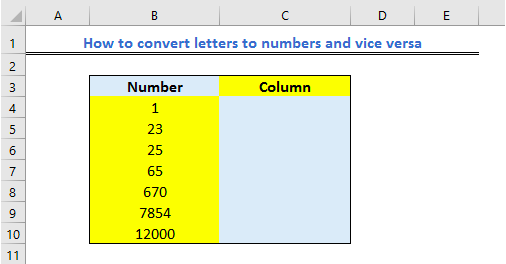Figure 16 – Setting up data for converting number to letter in excel

• We will press ALT + F11 to open the Microsoft Visual Basic Applications window
• Next, we will go to the Insert tab and Select Module
• In the blank space, we will enter the formula below

`Public Function ToColletter(Collet)`

`    ToColletter = Split(Cells(1, Collet).Address, "\$")(1)`

`End Function`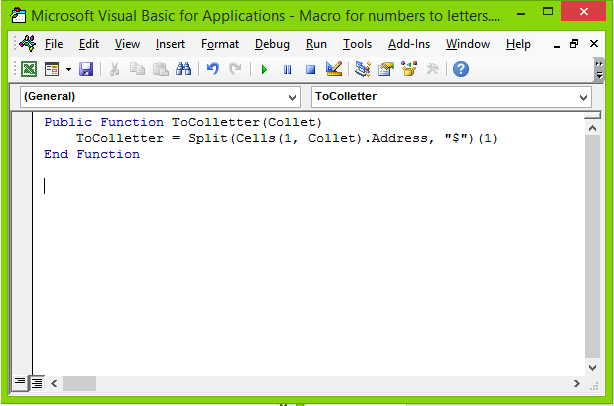Figure 17 – Excel Column number to letter using VBA

• Next, we will go back to the worksheet and enter the formula below in Cell C4

`=Tocolnum("1")`

Where 1 is the content of Cell B4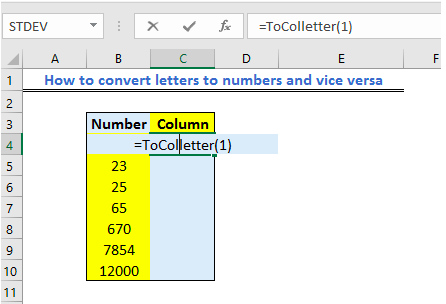Figure 18 – Convert column number to letter using VBA

• We will press the enter key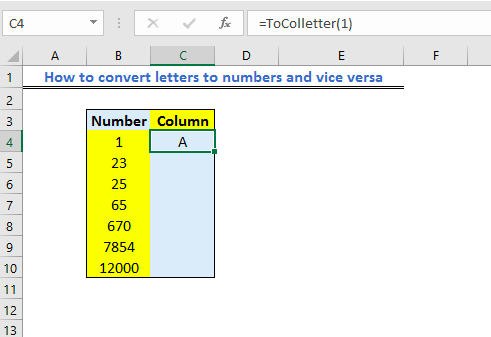Figure 19 – Excel convert column number to letter

• Again, to convert Cell B5, we will enter the formula below into Cell C5

`=ToColletter(23) `

## Where 23 is the content of Cell B5

• We will press the enter key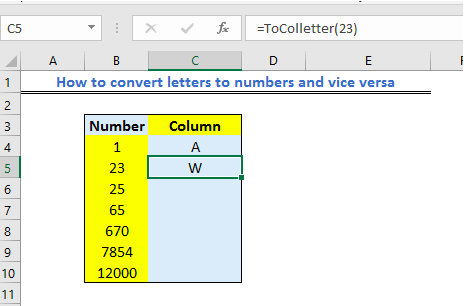Figure 20 – Convert letter to a number

• In the end, we will have this result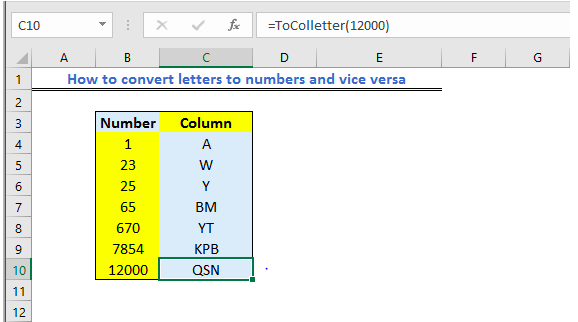Figure 21 – VBA for column number to letter

## Instant Connection to an Excel Expert

Most of the time, the problem you will need to solve will be more complex than a simple application of a formula or function. If you want to save hours of research and frustration, try our live Excelchat service! Our Excel Experts are available 24/7 to answer any Excel question you may have. We guarantee a connection within 30 seconds and a customized solution within 20 minutes.